﻿

### New generalizations of lifting modules

#### Abstract

In this paper, we call a module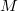$M$ almost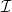$\mathcal{I}$-lifting if, for any element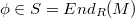$\phi\in S=End_R(M)$, there exists a decomposition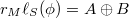$r_M\ell_S(\phi)=A\oplus B$ such that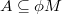$A\subseteq \phi M$ and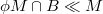$\phi M\cap B\ll M$. This definition generalizes the lifting modules and left generalized semiregular rings. Some properties of these modules are investigated. We show that if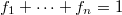$f_1+\cdots + f_n=1$ in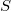$S$, where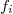$f_i$${^{ ,}}$s are orthogonal central idempotents, then$M$ is an almost$\mathcal{I}$-lifting module if and only if each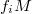$f_iM$ is almost$\mathcal{I}$-lifting. In addition, we call a module$M$$\pi$-$\mathcal{I}$-lifting if, for any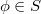$\phi\in S$, there exists a decomposition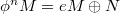$\phi^nM=eM\oplus N$ for some positive integer$n$ such that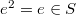$e^2=e\in S$ and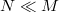$N\ll M$. We characterize semi-$\pi$-regular rings in terms of$\pi$-$\mathcal{I}$-lifting modules. Moreover, we show that if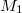$M_1$ and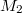$M_2$ are abelian$\pi$-$\mathcal{I}$-lifting modules with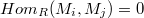$Hom_R(M_i, M_j)=0$ for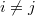$i\neq j$, then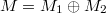$M=M_1\oplus M_2$ is a$\pi$-$\mathcal{I}$-lifting module.

DOI Code: 10.1285/i15900932v36n2p49

Keywords: Lifting module; $\mathcal{I}$-Lifting module; Semiregular ring; Semi-$\pi$-regular ring

Full Text: PDF